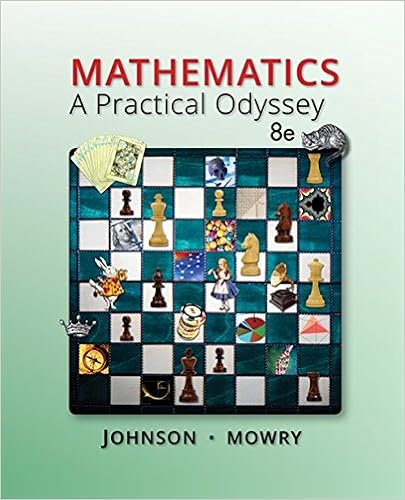# Free cash flow to base assumptions shares outstanding

• Test Prep
• 367
• 88% (17) 15 out of 17 people found this document helpful

This preview shows page 157 - 160 out of 367 pages.

##### We have textbook solutions for you!
The document you are viewing contains questions related to this textbook.The document you are viewing contains questions related to this textbook.
Chapter 11 / Exercise 5
Mathematics: A Practical Odyssey
Johnson/MowryExpert Verified
FREE CASH FLOW TO EQUITY
##### We have textbook solutions for you!
The document you are viewing contains questions related to this textbook.The document you are viewing contains questions related to this textbook.
Chapter 11 / Exercise 5
Mathematics: A Practical Odyssey
Johnson/MowryExpert Verified
15 - 32 Base Assumptions Shares outstanding (millions) 84 Required return on equity (r) 14% Projected 2001 Projected 2002 Projected 2003 Growth rate (g) 27% 27% 13% Total Per share Earnings after tax \$80 \$0.952 \$1.2090 \$1.5355 \$1.7351 Plus: Depreciation expense \$23 \$0.274 \$0.3480 \$0.4419 \$0.4994 Less: Capital expenditures \$38 \$0.452 \$0.5740 \$0.7290 \$0.8238 Less: increases in net working capital \$41 \$0.488 \$0.6198 \$0.7871 \$0.8894 Equals: FCFE \$24 \$0.286 \$0.3632 \$0.4613 \$0.5213 Terminal value \$52.1300* Total cash flows to equity \$0.3632 \$52.5910 ** Discounted value \$0.3186*** \$40.4673*** Current value per share \$40.7859 **** * Projected 2002 Terminal value = Projected 2003 FCFE/(r – g) **Projected 2002 Total cash flows to equity = Sum of Projected 2002 FCFE and Projected 2002 Terminal value *** Discounted values obtained using r = 14% **** Current value per share = Sum of Discounted Projected 2001 and 2002 Total cash flows to equity 26(c)i. The following limitations of the dividend discount model (DDM) are addressed by the FCFE model. The DDM uses a strict definition of cash flows to equity, i.e., the expected dividends on the common stock. In fact, taken to its extreme, the DDM cannot be used to estimate the value of a stock that pays no dividends. The FCFE model expands the definition of cash flows to include the balance of residual cash flows after all financial obligations and investment needs have been met. Thus the FCFE model explicitly recognizes the firm’s investment and financing policies as well as its dividend policy. In instances of a change of corporate control, and therefore the possibility of changing dividend policy, the FCFE model provides a better estimate of value. The DDM is biased toward finding low P/E ratio stocks with high dividend yields to be undervalued and
15 - 33 conversely, high P/E ratio stocks with low dividend yields to be overvalued. It is considered a conservative model in that it tends to identify fewer undervalued firms as market prices rise relative to fundamentals. The DDM does not allow for the potential tax disadvantage of high dividends relative to the capital gains achievable from retention of earnings. 26(c)ii. The following limitations of the DDM are not addressed by the FCFE model. Both two-stage valuation models allow for two distinct phases of growth, an initial finite period where the growth rate is abnormal, followed by a stable growth period that is expected to last “forever”. These two-stage models share the same limitations with respect to the growth assumptions. First, there is difficulty of defining the duration of the extraordinary growth period. For example, a longer period of high growth will lead to a higher valuation, and there is the temptation to assume an unrealistically long period of extraordinary growth. Second, the assumption of a sudden shift from high growth to lower, stable growth is unrealistic. Given that the assumed total horizon does not shift (i.e., is infinite), the timing of the shift from high to stable growth is a critical determinant of the valuation estimate. Third, because the value is quite sensitive to the steady-state growth assumption, over- or under-estimating this rate can lead to large errors in value.
•••